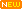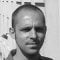Learn about power engineering and HV/MV/LV substations. Study specialized technical articles, electrical guides, and papers.

# Calculate Size of Diesel Generator

Home / Download Center / Electrical Software Reviews / Calculate Size of Diesel Generator

## Size of Diesel GenSet

This spreadsheet intend to calculate size of needed diesel generator according to the following input parameters:

1. System Voltage ( P-P ) in Volts
2. System Voltage ( P-N ) in Volts
3. Future Load Expansion in %
4. Average Intermittent Use of Equipment (0.7 To 1.0)

You are about to enter following information for each consumer you have in your consumption calculation:

1. Equipment (name)
2. Load Type (linear, non-linear or motor)
3. Supply (1ph or 3ph)
4. Qty (num.)
6. Starting P.F
7. Running P.F
8. Diversity Factor
9. Motor Starter (Dol, Y-D, auto-transformer, soft starter or frequency converter)

Calculated results consist of:

1. Size of Diesel GenSet for linear, Non-linear Load
2. Size of Diesel GenSet for motor load
3. Diesel GenSet Efficiency
4. UPL (unit per liter) of Diesel GenSet
5. Unit power cost of Diesel GenSet
6. Approximate fuel consumption
 Software: Calculate Size of Diesel Generator (MS Excel Spreadsheet) Version: 22.08.2012Developer: Jignesh Parmar Size: 138 Kb Price: Free Download: Right here | Video Courses | Membership | Download Updates

1.Fermin
May 11, 2018

Hi guys.
I am thinking to star a 3 phase motor 75 kw, 460 VAC with a Diesel generator (144 HP rated ), 460 VAC using a soft starter.
Sombody can help me if this is posible?

2.mina
Apr 08, 2018

hey guys

how can i calculate the Diversity Factor in a utility with 3 3ph motors that are working simultaneously?

•Mansour Essaïh
Oct 24, 2020

Better late than never.
The 3 mortors are working simultaneously, so the diversity factor Kd = 1 (*)

If ypu want to estimate the energy consumption per day, you have to use loading factor or utilization factor generaly = 0.80. But if you know the power absorbed by the machine driven by the electric motor, then, the utilization factor Ku = Machine power/Nominal motor power.

The global factor Kg = KdxKu.

If Ku = 0.8, then Kg = 1×0.8 = 0.8.

If the unit nominal power of your motors is Pn = 22 kW, the total power absorbed is : 3×0.8x 22 = 52.8 kW.

If your 3 motors work 8 hours per day, then the energy consumed per day is : E = NxKgxPnxt = 3×0.80x22x8 = 422.4 kWh.

3.vijay
Feb 08, 2018

Calculations shown for DG sizing is having minor errors, starting KVA is multiplied twice.

4.ahmed emara
Nov 10, 2016

Hey guys

now I have 1200 ekw generator with fuel consumption 333 l/hr and I need to calculate the efficiency of that generator set

is the efficiency subjected to the following equation ?:
Qin =Wout+Qrej

Then ᶮ ( efficiency ) = Wout/ Qin .. Wout = 1200 Kw
Where Qin = m°f *Cvf
Cvf ( for diesel) = 46000000 j/kg
ρ= 850 Kg/m³
But the result was not good

Regards

5.John Alias
Mar 10, 2016

can anyone please send the detailed calculations and formulas used in this spreadsheet?…it would be really helpful
Thank you

6.SHANTANU DESHPANDE
Oct 19, 2015

What is the difference between Average Intermittent Use and Diversity Factor ??

7.Faihan
Aug 24, 2015

Good Job
How do I add the Air Conditioning units? Are they linear or non? I only know the ton value of each.
And, What is the correct connection for single phase ACs and lights? Is it Y-D?
I also have a three phase 60 HP submersible pump that sucks 150 Amps on each phase to start and then goes to 90 Amps to run. Your sheet says that the full load Amps is 38 while I see on the DG display 90 Amps on each of the three phases.

8.abel
Jul 29, 2015

What about considering non linear loads in %age of total load in sizing? The limits of such loads govern sizing if exceeded. Any international standards talks about this in sizing the Genset? How it will be applied in the calculation?

9.NEIL MAJUMDER
Apr 27, 2015

Consider a case where EDG is to be started on to a distribution transformer.How to size the DG set then?

10.sriram
Apr 12, 2015

we have total load in kw .how do we arrive at genset capacity??/

11.Jul 21, 2014

How can we conduct the load test of DG set at site?

12.Ahmed
Dec 18, 2013

Engine overload is 10% only not 130 to 150%.

It is depend for Standby application or Prime or base load .
It is better to check and alternator efficiency is about 93 to 96

13.eleng2007
Oct 09, 2013

how to calculate the required daily / monthly fuel tank of generator?

•Edvard
Oct 09, 2013

Hooh, sounds very interesting, but I wouldn’t know really. I guess that eash manufacturer has its own manual or guide you should follow.

Anyway, your question’s answer depends on how many hours generator will work.

•Nov 23, 2014

14.srikanth.shanky
Aug 13, 2013

Wonder that the starting power factor of the motor assumed to be as 0.8 is correct.Normally the value may be 0.2-0.3.The pf at 80% of speed during starting may be 0.6.Moreover the highest rated motor starting with base load and as well as voltage drop need to be considered for DG sizing.

15.partoshal
Mar 25, 2013

Thanks a lot

•Edvard
Mar 25, 2013

Link works quite well, I double checked. Maybe you’re having problems with Box.com on your side?

16.Mahesh
Feb 26, 2013

17.JaymzSchmerzen
Jan 23, 2013

Real useful.. Double checked my calcs =)

Thanks a lot

18.Jerome
Jan 14, 2013

Sir,

How accurate the spreed sheet is? this have been tested on site?

Thanks!